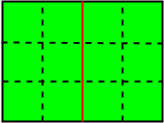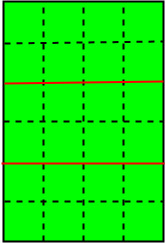# Maximum of smallest possible area that can get with exactly k cut of given rectangular

Given n×m big rectangular area with unit squares and k times cut is allowed, the cut should be straight (horizontal or vertical) and should go along the edges of the unit square. What is the maximum possible area of the smallest piece he can get with exactly k cuts.

Examples :

```Input : 3 4 1
Output : 6

Input : 6 4 2
Output : 8
```Image for 2nd inputImage for 1st input

## Recommended: Please try your approach on {IDE} first, before moving on to the solution.

As this is n×m rectangular area so there are (n-1) rows and (m-1) columns . So if k > (n + m – 2) then, then cut are not possible . Then, if k is less than that . There will be two cases

1. When k is less than max( n, m ) – 1 : In the 1st case, if k is less than max( n, m ) – 1, then either m * ( n / ( k+1 ) ) or n * ( m / ( k+1 ) ) is maximum, here we have divided by ( k + 1) because horizontally or vertically i.e ( m * n = total blocks ) is divided into ( k + 1 ) parts.
2. When k is greater than or equal to max( n, m) – 1 : In the 2nd case, if k >= max( n, m ) – 1, then there will be cut on both rows as well as columns so, the maximum possible smallest area will be either m / ( k – n + 2) or n / ( k – m + 2 ) . In this case suppose if n > m then, firstly n-1 (row or column) cut is possible . After that (k – n) cut will be done on m – 1 . So, here we have adjusted this ( k – n ) cut such that smallest possible division should be maximum.

Code – Below is the implementation of the following approach

## C++

 `#include ` `using` `namespace` `std; ` ` `  `void` `max_area(``int` `n, ``int` `m, ``int` `k) ` `{ ` `    ``if` `(k > (n + m - 2)) ` ` `  `        ``cout << ``"Not possible"` `<< endl; ` ` `  `    ``else` `{ ` ` `  `        ``int` `result; ` ` `  `        ``// for the 1st case ` `        ``if` `(k < max(m, n) - 1) { ` ` `  `            ``result = max(m * (n / (k + 1)), n * (m / (k + 1))); ` `        ``} ` ` `  `        ``// for the second case ` `        ``else` `{ ` ` `  `            ``result = max(m / (k - n + 2), n / (k - m + 2)); ` `        ``} ` ` `  `        ``// print final result ` `        ``cout << result << endl; ` `    ``} ` `} ` ` `  `// driver code ` `int` `main() ` `{ ` ` `  `    ``int` `n = 3, m = 4, k = 1; ` `    ``max_area(n, m, k); ` `} `

## Java

 `// Java code for Maximum of smallest  ` `// possible area that can get with  ` `// exactly k cut of given rectangular ` ` `  `class` `GFG { ` `     `  `    ``//Utility Function ` `    ``static` `void` `max_area(``int` `n, ``int` `m, ``int` `k) ` `    ``{ ` `        ``if` `(k > (n + m - ``2``)) ` `     `  `            ``System.out.println(``"Not possible"``); ` `     `  `        ``else` `{ ` `     `  `            ``int` `result; ` `     `  `            ``// for the 1st case ` `            ``if` `(k < Math.max(m, n) - ``1``)  ` `            ``{ ` `                ``result = Math.max(m * (n / (k + ``1``)),  ` `                         ``n * (m / (k + ``1``))); ` `            ``} ` `     `  `            ``// for the second case ` `            ``else` `{ ` `     `  `                ``result = Math.max(m / (k - n + ``2``),  ` `                         ``n / (k - m + ``2``)); ` `            ``} ` `     `  `            ``// print final result ` `            ``System.out.println(result); ` `        ``} ` `    ``} ` `     `  `    ``// Driver code ` `    ``public` `static` `void` `main (String[] args)  ` `    ``{ ` `        ``int` `n = ``3``, m = ``4``, k = ``1``; ` `        ``max_area(n, m, k); ` `    ``} ` `} ` ` `  `// This code is contributed by Anant Agarwal. `

## Python3

 `# Python3 code for Maximum of smallest  ` `# possible area that can get with  ` `# exactly k cut of given rectangular ` ` `  `def` `max_area(n,m,k): ` `    ``if` `(k > (n ``+` `m ``-` `2``)): ` `        ``print``(``"Not possible"``) ` `    ``else``: ` `        ``# for the 1st case ` `        ``if` `(k < ``max``(m,n) ``-` `1``):  ` `            ``result ``=` `max``(m ``*` `(n ``/` `(k ``+` `1``)), n ``*` `(m ``/` `(k ``+` `1``))); ` ` `  `        ``# for the second case ` `        ``else``: ` `            ``result ``=` `max``(m ``/` `(k ``-` `n ``+` `2``), n ``/` `(k ``-` `m ``+` `2``)); ` ` `  `        ``# print final result ` `        ``print``(result) ` ` `  `# driver code ` `n ``=` `3` `m ``=` `4` `k ``=` `1` ` `  `max_area(n, m, k) ` ` `  `# This code is contributed ` `# by Azkia Anam. `

## C#

 `// C# code for Maximum of smallest  ` `// possible area that can get with  ` `// exactly k cut of given rectangular ` `using` `System; ` ` `  `class` `GFG { ` `     `  `    ``//Utility Function ` `    ``static` `void` `max_area(``int` `n, ``int` `m, ``int` `k) ` `    ``{ ` `        ``if` `(k > (n + m - 2)) ` `     `  `            ``Console.WriteLine(``"Not possible"``); ` `     `  `        ``else` `{ ` `     `  `            ``int` `result; ` `     `  `            ``// for the 1st case ` `            ``if` `(k < Math.Max(m, n) - 1)  ` `            ``{ ` `                ``result = Math.Max(m * (n / (k + 1)),  ` `                        ``n * (m / (k + 1))); ` `            ``} ` `     `  `            ``// for the second case ` `            ``else` `{ ` `     `  `                ``result = Math.Max(m / (k - n + 2),  ` `                        ``n / (k - m + 2)); ` `            ``} ` `     `  `            ``// print final result ` `            ``Console.WriteLine(result); ` `        ``} ` `    ``} ` `     `  `    ``// Driver code ` `    ``public` `static` `void` `Main ()  ` `    ``{ ` `        ``int` `n = 3, m = 4, k = 1; ` `         `  `        ``max_area(n, m, k); ` `    ``} ` `} ` ` `  `// This code is contributed by vt_m. `

## PHP

 ` (``\$n` `+ ``\$m` `- 2)) ` ` `  `        ``echo` `"Not possible"` `,``"\n"``; ` ` `  `    ``else`  `    ``{ ` `        ``\$result``; ` ` `  `        ``// for the 1st case ` `        ``if` `(``\$k` `< max(``\$m``, ``\$n``) - 1)  ` `        ``{ ` ` `  `            ``\$result` `= max(``\$m` `* (``\$n` `/ (``\$k` `+ 1)),  ` `                          ``\$n` `* (``\$m` `/ (``\$k` `+ 1))); ` `        ``} ` ` `  `        ``// for the second case ` `        ``else`  `        ``{ ` ` `  `            ``\$result` `= max(``\$m` `/ (``\$k` `- ``\$n` `+ 2),  ` `                          ``\$n` `/ (``\$k` `- ``\$m` `+ 2)); ` `        ``} ` ` `  `        ``// print final result ` `    ``echo` `\$result` `,``"\n"``; ` `    ``} ` `} ` ` `  `// Driver Code ` `\$n` `= 3; ``\$m` `= 4; ``\$k` `= 1; ` `max_area(``\$n``, ``\$m``, ``\$k``); ` ` `  `// This code is contributed by ajit ` `?> `

Output :

```6
```

Don’t stop now and take your learning to the next level. Learn all the important concepts of Data Structures and Algorithms with the help of the most trusted course: DSA Self Paced. Become industry ready at a student-friendly price.

My Personal Notes arrow_drop_upCheck out this Author's contributed articles.

If you like GeeksforGeeks and would like to contribute, you can also write an article using contribute.geeksforgeeks.org or mail your article to contribute@geeksforgeeks.org. See your article appearing on the GeeksforGeeks main page and help other Geeks.

Please Improve this article if you find anything incorrect by clicking on the "Improve Article" button below.

Improved By : jit_t, sanskar27jain